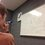# The Golden Ratio: Beginning the Algebra of

Here is the previous post concerning the Golden Ratio. For a collection of all the posts concerning the Golden Ratio, click #GoldenRatio below.

Today we start delving into the algebra around the golden ratio. Remember that the golden ratio is derived from the equation

$\phi^2=\phi +1$

And is equal to $\frac{1+\sqrt{5}}{2}$. Also, remember that we touched base a little on some interesting identities in one of the first posts. They were:

$\phi=\sqrt{1+\sqrt{1+\sqrt{1+\sqrt{1+\sqrt{1+\cdots}}}}}$

$\phi=1+\frac{1}{1+\frac{1}{1+\frac{1}{1+\frac{1}{1+\cdots}}}}$

To move on, we need to first introduce Fibonacci numbers. But before we do this, I have a challenge for you. We already know that $\frac{1+\sqrt{5}}{2}$ is equal to the golden ratio, but can you express $\frac{1-\sqrt{5}}{2}$, $\frac{-1+\sqrt{5}}{2}$, and $\frac{-1-\sqrt{5}}{2}$ in terms of the golden ratio? Post your answers and work below.Note by Bob Krueger
7 years, 4 months ago

This discussion board is a place to discuss our Daily Challenges and the math and science related to those challenges. Explanations are more than just a solution — they should explain the steps and thinking strategies that you used to obtain the solution. Comments should further the discussion of math and science.

When posting on Brilliant:

• Use the emojis to react to an explanation, whether you're congratulating a job well done , or just really confused .
• Ask specific questions about the challenge or the steps in somebody's explanation. Well-posed questions can add a lot to the discussion, but posting "I don't understand!" doesn't help anyone.
• Try to contribute something new to the discussion, whether it is an extension, generalization or other idea related to the challenge.
• Stay on topic — we're all here to learn more about math and science, not to hear about your favorite get-rich-quick scheme or current world events.

MarkdownAppears as
*italics* or _italics_ italics
**bold** or __bold__ bold
- bulleted- list
• bulleted
• list
1. numbered2. list
1. numbered
2. list
Note: you must add a full line of space before and after lists for them to show up correctly
paragraph 1paragraph 2

paragraph 1

paragraph 2

[example link](https://brilliant.org)example link
> This is a quote
This is a quote
    # I indented these lines
# 4 spaces, and now they show
# up as a code block.

print "hello world"
# I indented these lines
# 4 spaces, and now they show
# up as a code block.

print "hello world"
MathAppears as
Remember to wrap math in $$ ... $$ or $ ... $ to ensure proper formatting.
2 \times 3 $2 \times 3$
2^{34} $2^{34}$
a_{i-1} $a_{i-1}$
\frac{2}{3} $\frac{2}{3}$
\sqrt{2} $\sqrt{2}$
\sum_{i=1}^3 $\sum_{i=1}^3$
\sin \theta $\sin \theta$
\boxed{123} $\boxed{123}$

## Comments

Sort by:

Top Newest

Can an admin edit this note so that the tags #CosinesGroup, #GoldenRatio, and #Algebra appear?

- 7 years, 4 months ago

Log in to reply

That wasn't hard.

$\frac{1 - \sqrt{5}}{2} = 1 - \phi = -\frac{1}{\phi} = - \cfrac{1}{1+\cfrac{1}{1+\cfrac{1}{(\cdots)}}}$ $\frac{-1 + \sqrt{5}}{2} = \phi - 1 = \frac{1}{\phi} = \cfrac{1}{1+\cfrac{1}{1+\cfrac{1}{(\cdots)}}}$ $\frac{-1 - \sqrt{5}}{2} = - \phi = -1 - \cfrac{1}{1+\cfrac{1}{1+\cfrac{1}{(\cdots)}}}$

PS: This is what I was doing before going to sleep... I literally did the same exercises :D

- 7 years, 4 months ago

Log in to reply

×

Problem Loading...

Note Loading...

Set Loading...